output.to from Sideway
Algebra

Draft for Information Only

# Content

```  Sequence of First n Natural Numbers   First n Natural Numbers Series   Sequence of First n Natural Number Squares[1,2]   First n Natural Number Squares Series[1,2]   Sequence of First n Natural Number Cubes[1,2]   First n Natural Number Cubes Series[1,2]```

## Sequence of First n Natural Numbers

The sequence of the first n natrual numbers  are, 1, 2, 3, ...., n. The first n natural numbers sequence is an ordered arithmetic sequence with initial term equal to 1 and common difference equal to 1. Imply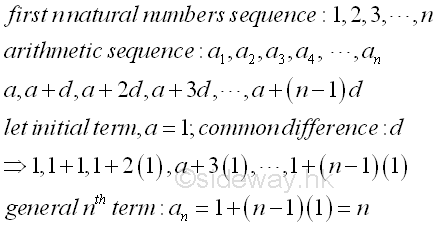### First n Natural Numbers Series

Since the first n natural number is an arithmetic sequence, the series of the first n natural numbers can also be determined as an arithmetic series. As an arithmetic series is of the form S=a+(a+d)+(a+2d)+(a+3d)+.... And the general format of an arithmetic series is Sn=a1+a2+a3+a4+ ....+an-1+an =a+(a+d)+(a+2d)+(a+3d)+...+(a+(n-2)d)+(a+(n-1)d) =∑ n
i=1
(ai) =∑ n-1
k=0
(a+kd) =1+2+3+4+ ....+(n-1)+n . The sum of an arithmetic series can be determined by the adding the reverse order of the arithmetic series to the original arithmetic series, so that the sum of two arithmetic series can be expressed as the sum of  n equal terms of first term plus last term. And the sum of arithmetic series is therefore equal to n(2a+(n-1)d)/2=(n(n+1))/2. Imply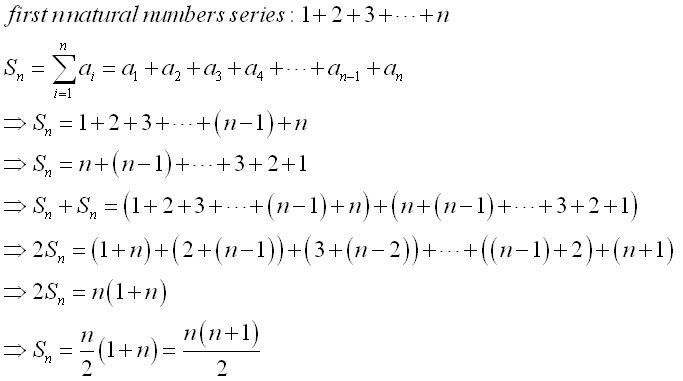### Sequence of First n Natural Number Squares[1,2]

The sequence of the first n natrual numbers squaes are, 1, 4, 9, ...., or 1, 22, 32, ...., n2. The first n natural number squares sequence is an ordered sequence with initial term equal to 1 and variable difference of two consecutive number squares equal to k2-(k-1)2 =2k-1. That is 1, 1+(2(2)-1), 1+(2(2)-1)+(2(3)-1), 1+(2(2)-1)+(2(3)-1)+(2(4)-1),... or  1, 1+3, 1+3+5, 1+3+5+7,...,1+3+5+7+...+(2n-3)+(2n-1). Therefore the general nth term is equal to an=n2=∑ n
i=1
(2i-1).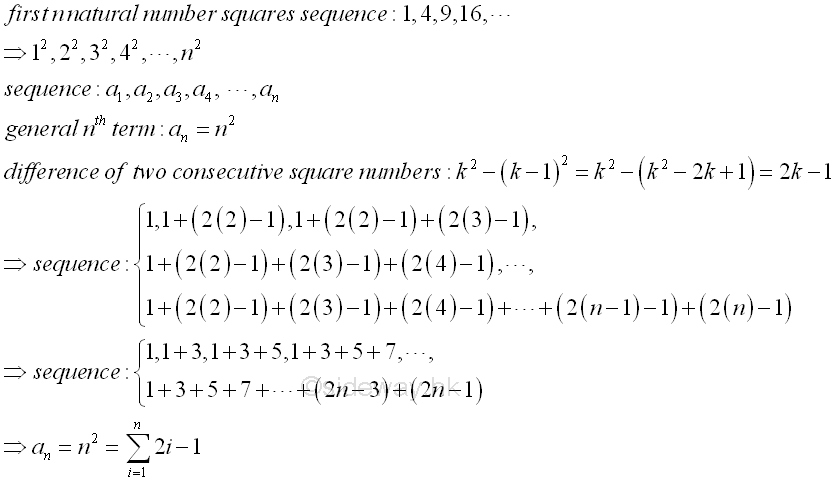### First n Natural Number Squares Series[1,2]

Since the common difference varies with the term, the sum of the sequence cannot be determined by the method of finding the sum of an arithmetic series directly. How the technique used to determine the sum of the first n natural number squares series is similarly. First, since the series of natural number squares has a variable difference equal to 2k-1, each term of the series can be expressed as a series, and the natural number since can be written as 1+(1+3)+(1+3+5)+(1+3+5+7+9)+...+(1+3+5+7+9+...+2n-3)+(1+3+5+7+9+...+2n-3+2n-1).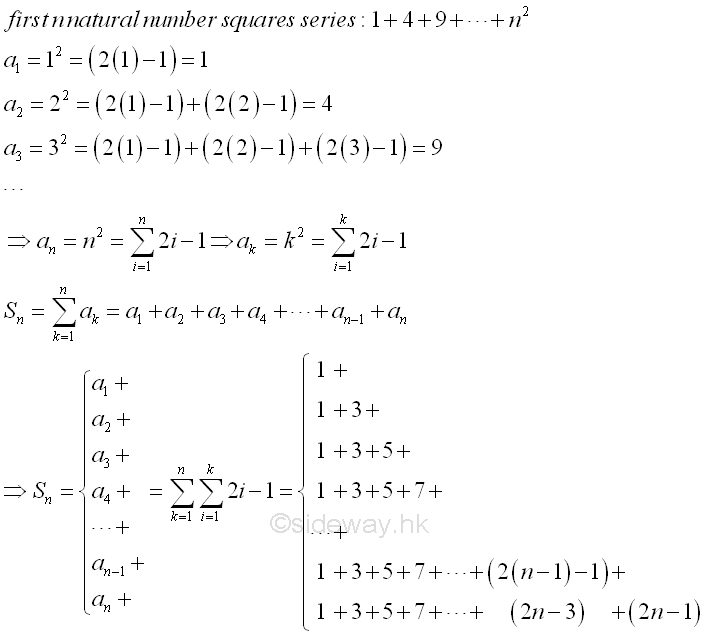Second, apply the technique used in determining the sum of an arithmetic series, each series term of the series can be added to the reverse order of the series term itself, so that the sum of two natural number squares can be expressed in term of the sum of the first and last terms times the item number also. That is (1+1)+((1+3)+(3+1))+((1+5)+(3+3)+(5+1))+...+((1+(2n-1))+(3+(2n-3))+...+((2n-3)+3)+((2n-1)+1)) =(2)+(4+4)+(6+6+6)+...+(2n+2n+...+2n+2n).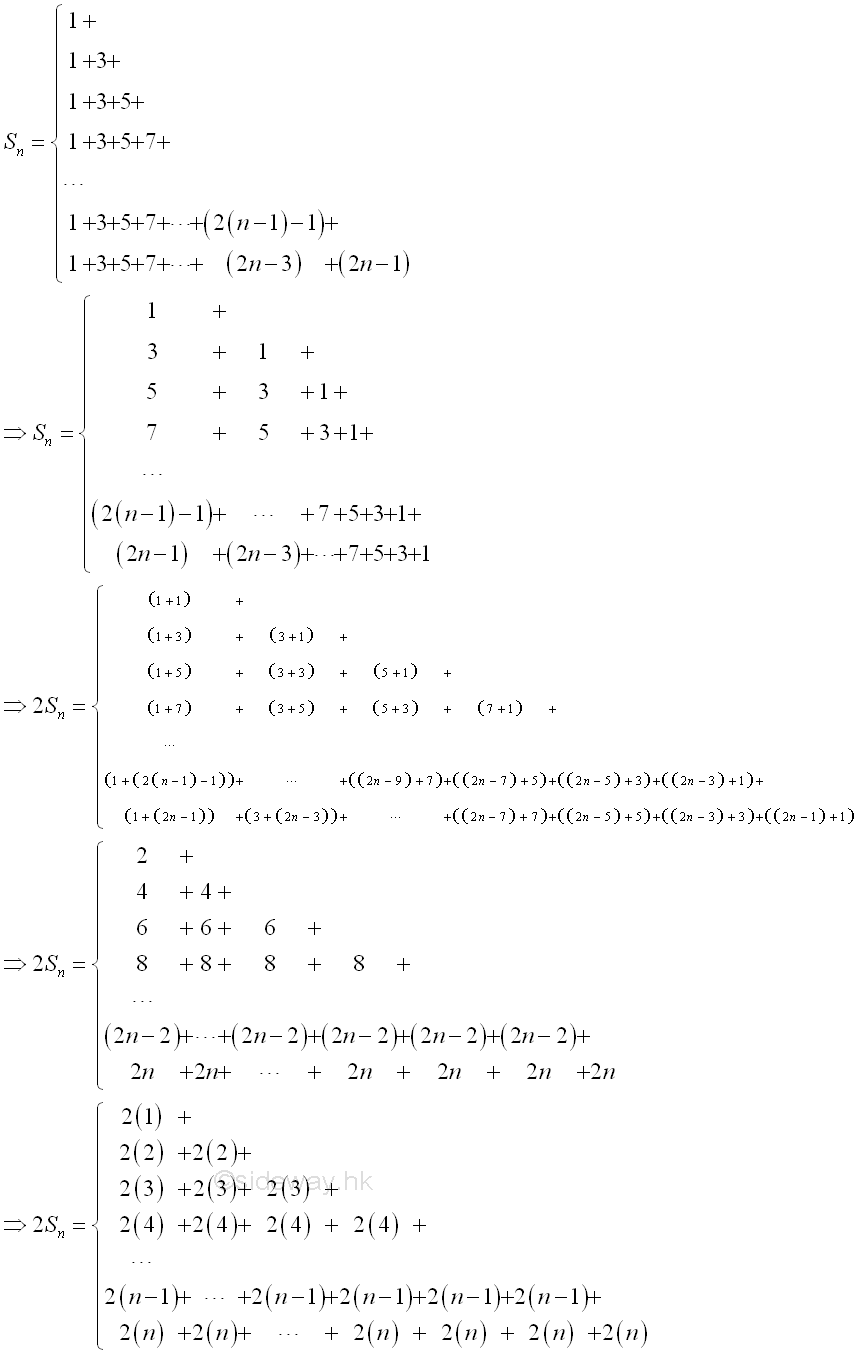Third, by comparing the new series of the sum of two series and the series of the original natural number squares series, there is similarity between the two series. For the original series, the total number of the same series item in the whole series number is in reversed order of the term number, i.e. n items of 1, n-1 items of 3, n-2 items of 5, ..., 1 item of 2n-1. But for the new series of the sum of two series, the total number of the series item is of the same order of the term item, i.e.  n items of 2n, n-1 items of 2(n-1) n-2 items of 2(n-2), ...,  3 items of 2(3), 2 items of 2(2), 1 items of 2(1). Finally, when all paired terms with equal numbers of items in the two series are added together, the sums of all paired terms are equal to 2n+1. In other words, the sum of two series becomes an arithmetic series with initial term 2n+1 and common difference 2n+1 with the first term equal to 2n+1 and the last term equal to n(2n+1). For an arithmetic series, the sum of the two series is equal to (n(n(2n+1)+(2n+1)))/2=(n(n+1)(2n+1))/2. Since the sum is equal to three original natural number square series, the sum of one natural number squares series is therefore equal to (n(n+1)(2n+1))/6. Imply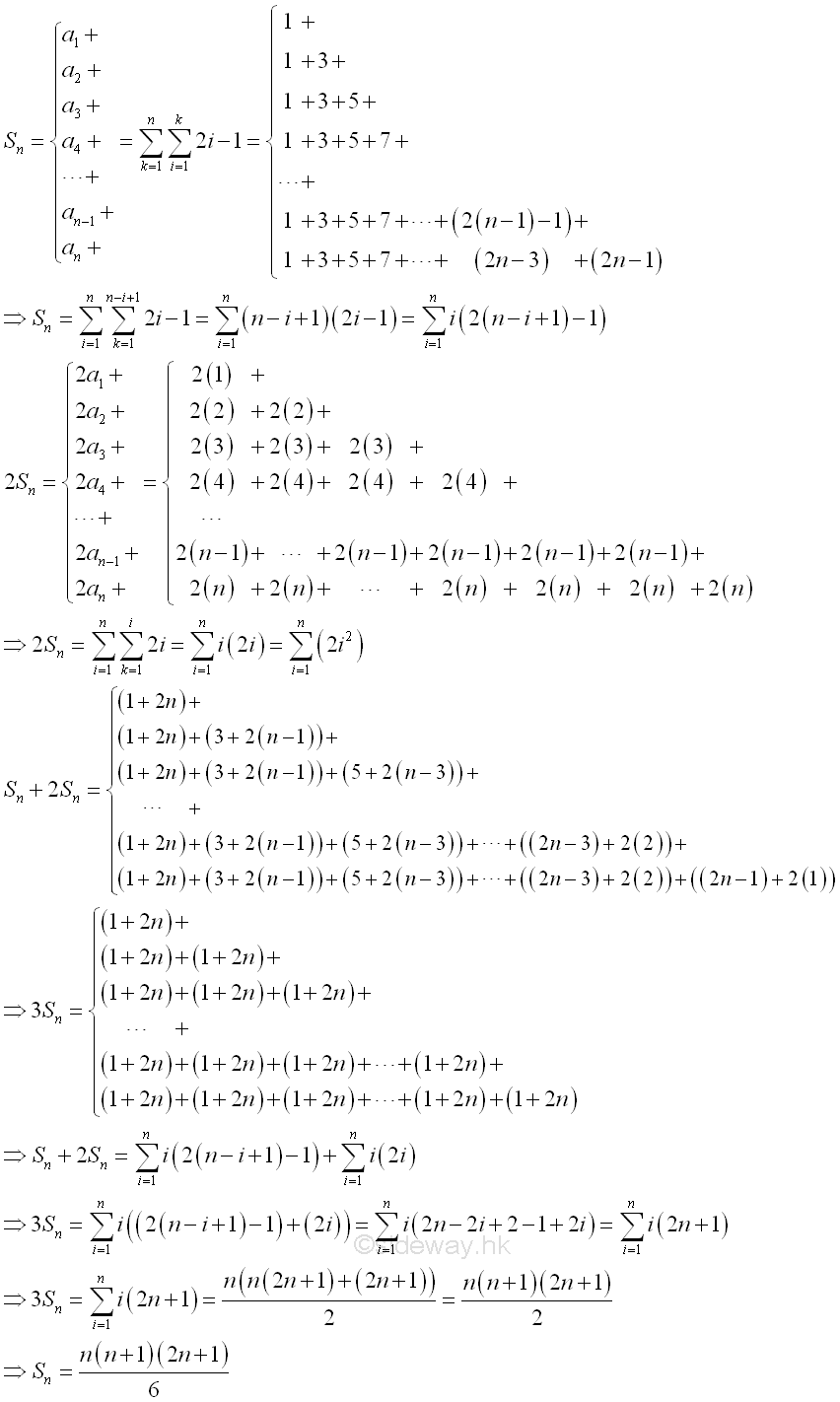### Sequence of First n Natural Number Cubes[1,2]

The sequence of the first n natrual numbers cubes are, 1, 8, 27, ...., or 1, 23, 33, ...., n3. The first n natural number cubes sequence is an ordered sequence with initial term equal to 1 and variable difference of two consecutive number squares equal to k3-(k-1)3 =3k2-3k+1. That is 1, 1+(3(2)2-3(2)+1), 1+(3(2)2-3(2)+1)+(3(3)2-3(3)+1), 1+(3(2)2-3(2)+1)+(3(3)2-3(3)+1)+(3(2)2-3(2)+1),... or  1, 1+7, 1+7+19, 1+7+19+37,...,1+7+19+37+...+(3n2-9n+7)+(3n2-3n+1). Therefore the general nth term is equal to an=n3=∑ n
i=1
(3i2-3i+1).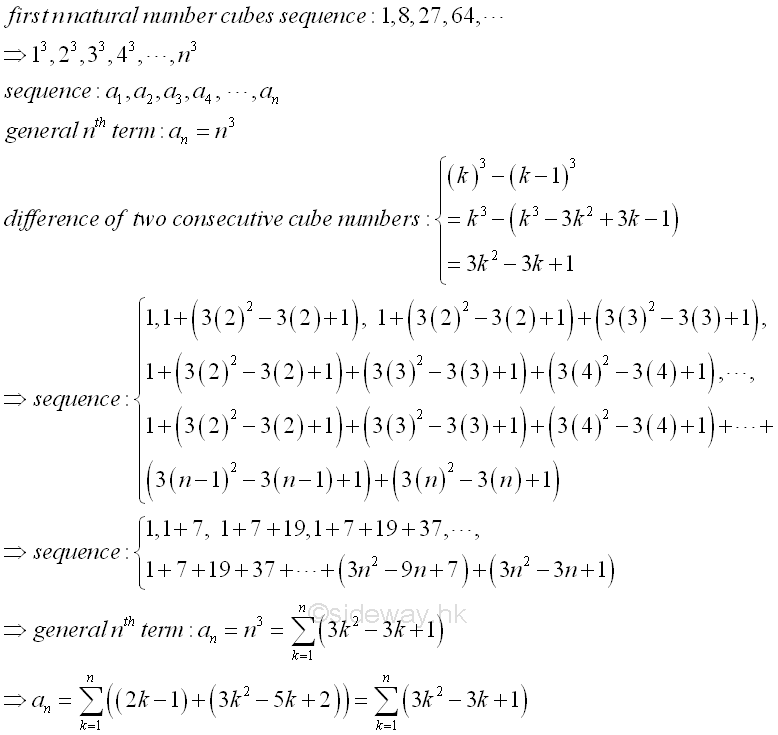### First n Natural Number Cubes Series[1,2]

As in natural number squares series, since the common difference varies with the term, the sum of the sequence cannot be determined by the method of finding the sum of an arithmetic series directly. However, the natural number cubes can also be expressed in a more strategic way such that the sum of the sequence can be found more easily. Instead of use a constant initial term, a variable initial term n2-n+1 can also be used so that n3 is equal to the sum of an arithmetical progression of n terms with common difference, 2. Therefore the sequence of natural number cubes can be rewritten as 1, 3+5, 7+9+11, 13+15+17+19,...,(n2-n+1)+(n2-n+1)+(n2-n+1)+(n2-n+3)+(n2-n+5)+...+(n2-n+2(n-1)-1)+(n2+n-1). And the general nth term is equal to an=n3= n
k=1
(n2-n+(2k-1))
.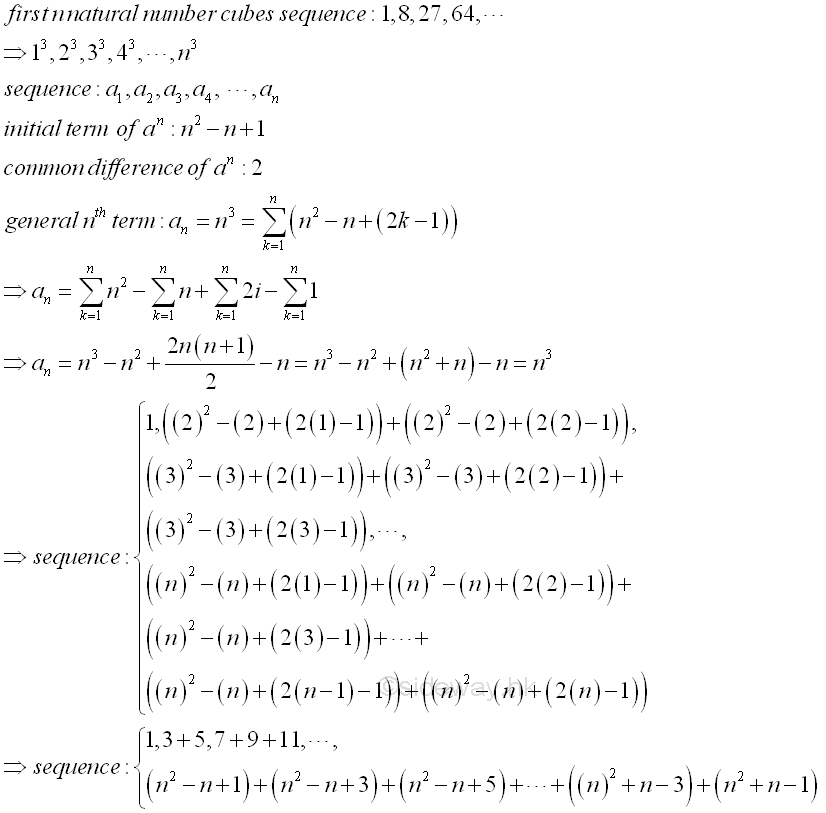The sequence becomes a triangular arrangement of odd numbers in a regular order. The ith cube is the sum of i number of consecutive odd number with initial term equal (i2-i+1)=i(i-1)+1. Therefore the sum of the natural number cubes series is equal to the sum of consecutive odd numbers with first term, 1 and last term, (n2+n-1). The total number of terms in the series is equal to (n(n+1))/2. That is 1+3+5+7+9+11+13+15+17+19+...+(n2+n-3)+(n2+n-1)= ∑ (n(n+1))/2
k=1
(2k-1). Since the sum of the natural number cubes series can be expressed in the form of an arithmetic series, the sum of natural number cubes is equal to (((n(n+1))/2)((n2+n-1)+1))/2=((n(n+1))/2)((n(n+1))/2)=((n(n+1))/2)2.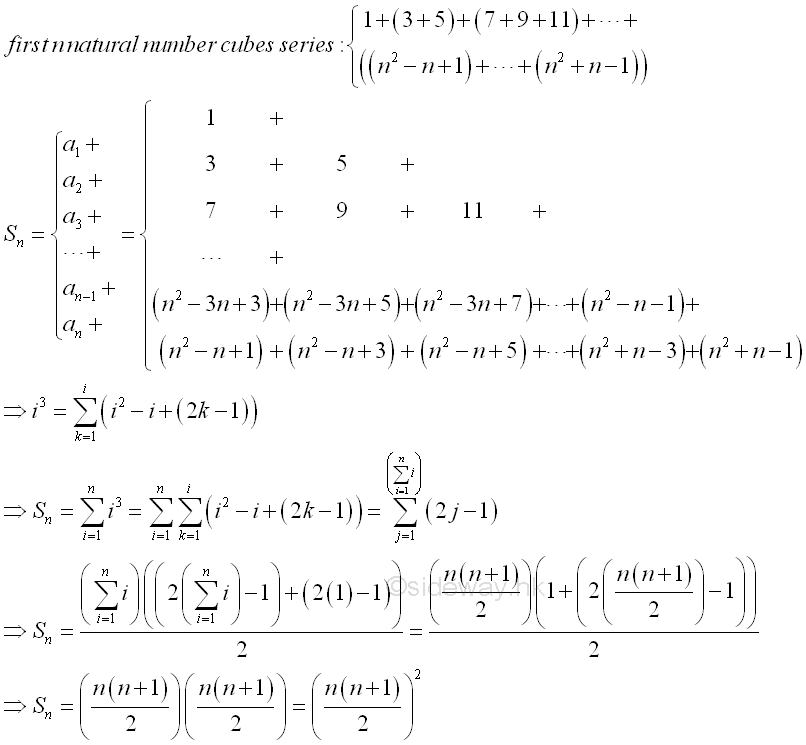©sidewayReferences

1. B. Joseph, 1978, University Mathematics: A Textbook for Students of Science & Engineering, Blackie & Son Limited, HongKong
2. Wheatstone, C., 1854, On the Formation of Powers from Arithmetical Progressions, Proceedings of The Royal Society of London, Vol 7, p145-151,, London
3. Stroud, K.A., 2001, Engineering Mathematics, Industrial Press, Inc, NY
4. Coolidge, J.L., 1949, The Story of The Binomial Theorem, The American Mathematical Monthly, Vol 56, No.3, Mar, pp147-157ID: 130500019 Last Updated: 2013/5/20 Revision: 1 Ref:Home (5)

Business

Management

HBR (3)

Information

Recreation

Hobbies (7)

Culture

Chinese (1097)

English (336)

Reference (66)

Computer

Hardware (149)

Software

Application (187)

Digitization (24)

Numeric (19)

Programming

Web (644)CSS (SC)

ASP.NET (SC)

HTML

Knowledge Base

Common Color (SC)

Html 401 Special (SC)

OS (389)MS Windows

Windows10 (SC)

.NET Framework (SC)

DeskTop (7)

Knowledge

Mathematics

Formulas (8)

Number Theory (206)

Algebra (20)

Trigonometry (18)

Geometry (18)

Calculus (67)

Complex Analysis (21)

Engineering

Tables (8)

Mechanical

Mechanics (1)

Rigid Bodies

Statics (92)

Dynamics (37)

Fluid (5)

Control

Acoustics (19)

Biology (1)

Geography (1)

Latest Updated Links

Copyright © 2000-2019 Sideway . All rights reserved Disclaimers last modified on 10 Feb 2019